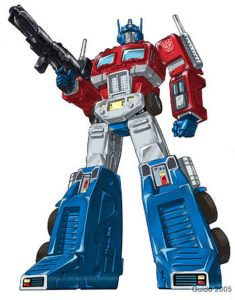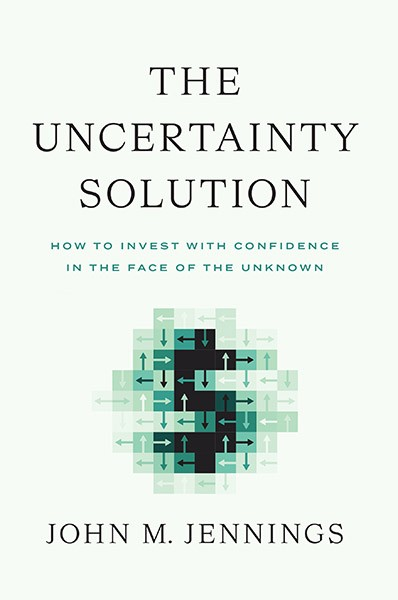## Good News – Prime Numbers Go All the Way Up!

by | Jul 24, 2017A prime number is a natural number greater than 1 that is divisible by itself and 1 and no other number.  Prime numbers include:

2, 3, 5, 7, 11, 13, 17, 19, 23, 29, 31, 37, 41, 43, 47, 53 . . . .

With the use of computers, prime numbers have been proven out to millions of digits. Earlier this year the largest prime of 274,207,281 − 1 was discovered. That’s two, multiplied 74,207,281 times, with 1 subtracted from it – it has 22,338,618 digits.  Currently, to discover a large prime like this it takes a computer running constantly over a month. The largest prime discovered without a computer was 44 digits in length and found in 1951.Even without proving larger and larger primes, we know that primes are infinite because of a proof by the ancient mathematician Euclid in about 300BC.  His proof of the infinitude of the primes is considered by some to be one of the most crucial pillars of human knowledge that has been ever been found and is a very beautiful piece of reasoning (a book I read a few years ago said that not knowing of this proof is a gap of experience in your life equivalent to having never tasted chocolate or having heard a single piece of music).   Below is the reasoning behind the proof – a bit wonky but does not require hardly any math knowledge to follow.

First, a basic rule of primes and numbers that is necessary to understand the proof:  Non-prime numbers must have some prime divisor.  In other words, every positive non-prime integer larger than one can be expressed as a product of one or more primes. (Go ahead and try this out: 4 is divisible by 2; 6 is divisible by 2 and 3; 8 by 2; 9 by 3; 10 by 2 and 5; 12 by 2 and 3; 14 by 2 and 7; 15 by 3 and 5; 16 by 2; 18 by 2 and 3; 20, by 2 and 5, and so on.)

The proof is as follows:  Assume that there is a Great Last Prime Number (i.e. if prime numbers are not infinite there must be a last one) and that the name of this Great Last Prime Number is “Optimus Prime.”*  For Optimus Prime to exist, there must be a finite, closed “Club” of all primes of which Optimus Prime itself is the final member (I would guess that the other primes would elect Optimus Prime an officer of the Club, maybe even president, but that is just conjecture on my part).

Suppose you multiply together every single member of the Club of all finite primes, including Optimus Prime.  You’ll get an absolutely GINORMOUS number that we can refer to as G (for Ginormous).  Because G is the product of all the primes in the finite club of primes, it can be divided by all the primes.  So, G is divisible by 2, 3, 5, 7, 11, etc.

Now consider the next integer in the number-line:  G + 1.  This number G + 1 cannot be a prime number because is way way way up the number-line from Optimus Prime, which, as you recall, is the last prime.  So, G + 1 has to have a prime divisor because of the rule stated previously that composite numbers must have a prime divisor (and if positive integer is not a prime number then it must be a non-prime).

What could that prime divisor of G + 1 be?  It can’t be 2 because G (which is one number below G + 1) is divisible by 2 and two even numbers obviously cannot be located next to each other (only even numbers are divisible by 2).  G + 1 cannot be divided by 3 because G is divisible by 3 and numbers divisible by 3 likewise are never next to each other (try it – count by three, the numbers are never next to each other).  Go on and on.  No matter what prime number you select (each of whom G is divisible by because G is the product of all the primes) you can’t divide G + 1 by that prime.  That is because whatever prime you pick divides G + 1’s lower neighbor, G, and multiples of whatever prime number you pick are never next door neighbors on the integer number-line.

Recall the rule that a non-prime number must be divisible by a prime number.  Thus, if G + 1 were to be a non-prime number then it must have a prime divisor, but as shown in the prior paragraph G + 1 is not divisible by any of the prime numbers in the Club of the finite prime numbers of which Optimus Prime is the last member.  Thus, there is a contradiction.  Optimus Prime is supposed to be the last prime, but G + 1 is not divisible by a prime so it must be a prime number and it is bigger than Optimus Prime.  Thus, Optimus Prime, the Great Last Prime cannot exist.  There cannot be a Club of finite primes.  With this logical proof, Euclid proved that prime numbers must be infinite.  Which is pretty cool.

Congrats if you read this whole thing and followed it. You are officially a nerd. Your nerd club card will be in the mail.

*Euclid didn’t actually call the Great Last Prime “Optimus Prime” – he called it “P.” Optimus Prime, of course, is a great robot warrior and one of the Transformers (pictured above).

# 1 Comment

1. I look forward receiving my Nerd Club card.

This site uses Akismet to reduce spam. Learn how your comment data is processed.## Archives## Subscribe To The IFOD

Get the Interesting Fact of the Day delivered twice a week. Plus, sign up today and get Chapter 2 of John's book The Uncertainty Solution to not only Think Better, but Live Better. Don't miss a single post!